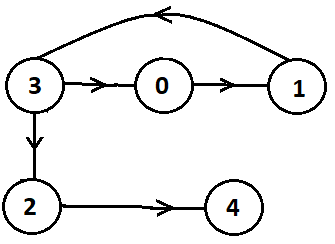New update is available. Click here to update.

# Safe Nodes In The Graph

Last Updated: 31 Mar, 2021
Difficulty: Moderate

## PROBLEM STATEMENT

#### For Example: For the graph, as shown in the picture below, [2, 4] are the only safe nodes.#### Ninja wants to know all the safe nodes in the graph in sorted order. Can you help Ninja to find out all the safe nodes?

##### Input Format
``````The first line of input contains an integer 'T' representing the number of test cases. Then the test cases follow.

The first line of each test case contains an integer ‘N’ and ‘E’ representing the number of nodes and edges in the graph.

The next ‘E’ lines of each test case contain two single space-separated integers denoting ‘EDGES[i]’ and ‘EDGES[i]’ which is a directed edge from node ‘EDGES[i]’ to ‘EDGE[i]’.
``````
##### Output Format :
``````For each test case, print the safe nodes in sorted order.

The output for each test case is printed in a separate line.
``````

#### Note:

``````You do not need to print anything; it has already been taken care of. Just implement the given function.
``````
##### Constraints :
``````1 <= T <= 100
1 <= N <= 10 ^ 4
1 <= E <= 10 ^ 4
0 <= EDGE[i] and EDGE[i] < N

Where ‘EDGE[i]’ and ‘EDGE[i]’ represents the directed edge.

Time Limit: 1 sec
``````## Approach 1

The idea behind this approach is we try to find if there is a cycle from the node we start. If we are able to find it, then we will mark that node and remove it, and if we cannot reach it, then after some number of steps, we'll stop.

A node will be ultimately safe if all of its outgoing edges to nodes are safe.

We can consider any node which is only pointing to safe nodes.

Then, we can update those nodes again, and so on, we do this for all the nodes.

Here is the complete algorithm:

• We will make a matrix/list ‘GRAPH’ using the given array/list ‘EDGES’.
• Insert all ‘EDGES[i]’ at index ‘EDGES[i]’ of the ‘GRAPH’.
• Make a queue 'QUE', an array/list ‘SAFE’ of size ‘N’ and arrays/lists ‘GRAPH1’ and ‘GRAPH2’ of type HashSet because the insertion and deletion operation in HashSet is of order O(1).
• ‘GRAPH1’ will store edges from ‘i’ to ‘j’.
• ‘GRAPH2’ will store edges from ‘j’ to ‘i’.
• Run a for loop from ‘i’ = 0 to ‘N’ and for each ‘i’ do the following:
• If the size of ‘GRAPH1[i]’ is zero. Then add ‘i’ to the 'QUE'.
• Traverse all the neighbors of the node ‘i’ do the following:
• Add neighbor to the ‘GRAPH1[i]’.
• Add node ‘i’ to the ‘GRAPH2[j]’.
• While 'QUE' is not empty do the following:
• Pop the node ‘temp’ from the 'QUE' and mark it visited.
• Traverse all the neighbors of the ‘temp’ in ‘GRAPH2’ and do the following:
• Remove the ‘temp’ node from the ‘GRAPH1’.
• If the size of ‘GRAPH1[neighbor]’ is zero
• Add neighbors to the 'QUE'.
• Traverse the ‘SAFE’ array/list if ‘SAFE[i]’ is true then include it in the final answer.